Table of Content

## Linear Regression

It is a type of predictive modelling technique based on statistics, created to predict the value of a specific entity based on historical data. It is a supervised machine learning algorithm. It is used to predict the value of a continuous variable also known as target variable or response variable based on one or more than one predictor (also known as features or independent variables).

## What is linear regression used for?

Linear regression can be used in different sectors viz. in real estate sector for the valuation of a property, in the retail sector for predicting monthly sales and the price of goods, for estimating the salary of an employee, in the educational sector for predicting the %marks of a student in the final exam based on his previous performance, etc. Financial forecasting is a classic application of regression that uses related information to predict the future value of entities like revenues, expenses, exchange rates, and capital costs.

## Why is linear regression a supervised machine learning algorithm ?

Linear regression is a supervised machine learning technique in which the system is trained with the target variable for identifying the trend before it can predict the outcome with unknown feature variable.

For example, consider the diagram below, in which a training model is constructed consisting of a single feature variable which is GRE score, and a target variable which is the % chance of getting admission into the University.

Once the machine is trained with the specified model, the system can predict the target variable (% change of getting admission) based on the test dataset containing unlabelled GRE score as specified in the diagram below.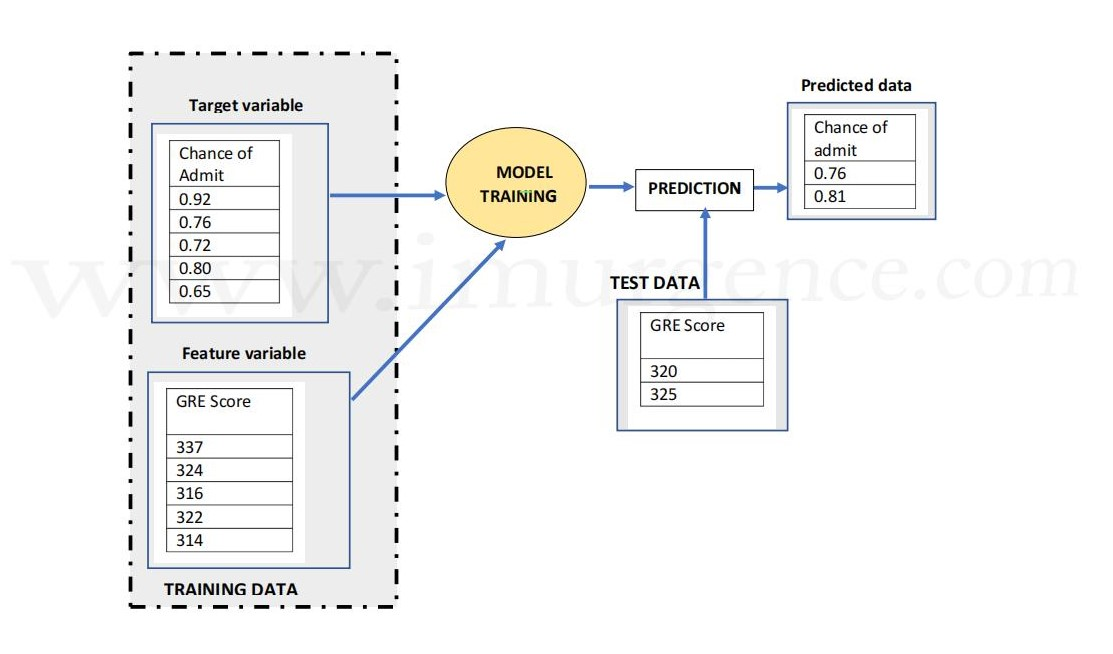Figure 1 : Linear Model predicting using Features and Target variable on Test Data

## What are the types of linear regression?

There are primarily two types of linear regression, simple linear regression and multiple linear regression.

### Simple Linear Regression

In simple linear regression a single feature variable or predictor determines the value of target variable or the outcome.

The equation is: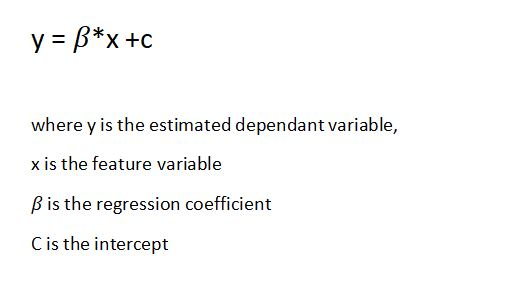## Multiple Linear Regression

In Multiple linear regression, multiple feature variables are involved in determining the outcome or the value of target variable.

The equation is as follows: -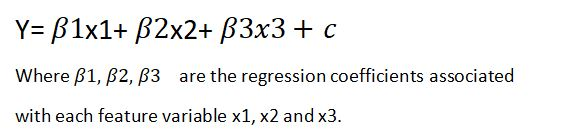## How do you calculate parameters of simple linear regression?

Linear regression models the linear relationship between two variables using a simple regression line which is a straight line. The two variables here are the independent variable, which is the cause and the dependant variable also known as the output, target or the response variable which depicts the effect.

For example, let us consider two variables which are linearly related. We need to find a linear function that predicts the response value(y) with the help of its feature variable or independent variable(x).

The simple linear regression equation with one dependent or feature variable and one independent or response variable is defined by the formula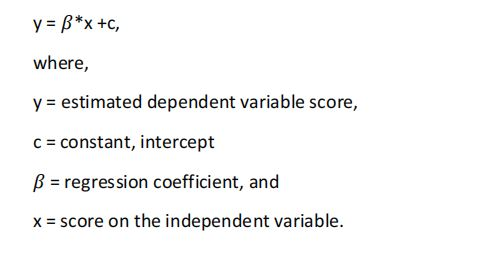We have a sample data set in which value of response y against every feature x is tabulated as in Figure 2:

x as feature vector
y as response vector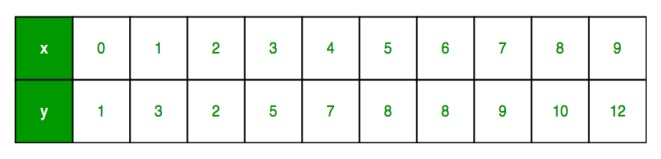Figure 2: Table of sample data

### Scatter plot

A scatter chart helps to visualize the response against every feature and we are trying to draw a line draw covering most of the data points. We can also call this as a regression line.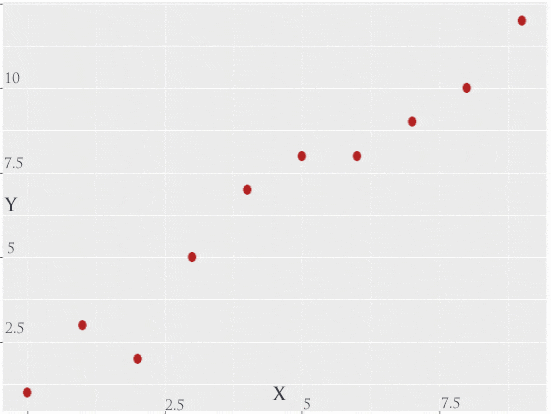Figure 3: Scatter Plot and Straight Linear Line Passing through data

## General trend of a linear regression line

A regression line is a straight line depicting the linear relationship between the two variables and is represented by a regression coefficient( ß) which is also the slope of the line, and the intercept which is also labelled as constant and is the expected mean value of y when all x=0.

There can be 3 different scenarios:
1. When there is no change in the value of y with respect to change in the value of x, ß is 0.
2. With increase in value of x, the value of y increases (x is directly proportional to y), ß is +ve.
3. With increase in value of x, y decreases (x is inversely proportional to y), ß is -ve.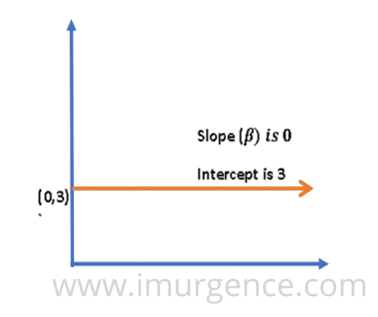Figure 4 : Trend of Linear Regression Line

## Case study (University admission prediction)

The University Admission Prediction dataset contains several parameters which are considered important during the application for Masters Programs. The data is available on kaggle. We have kept a copy of the University Admission Prediction dataset here.

For Simple linear regression let us consider the “GRE Score” (out of 340) as the feature which influences the “chance of admit” which ranges from 0 to 1.

### Step1: Plotting a scatter chart

Plot a scatter chart to analyse the relationship between the variables. Linear regression is possible only if a linear relationship between the two variables exists. In scatter chart the points should fall along a line and not be like a blob. We have taken only the first 5 records here for analysis.

GRE score Chance of admit
337
0.92
324
0.76
316
0.72
322
0.8
314
0.65

Figure 5 : Snapshot of top 5 Records in University Admission Dataset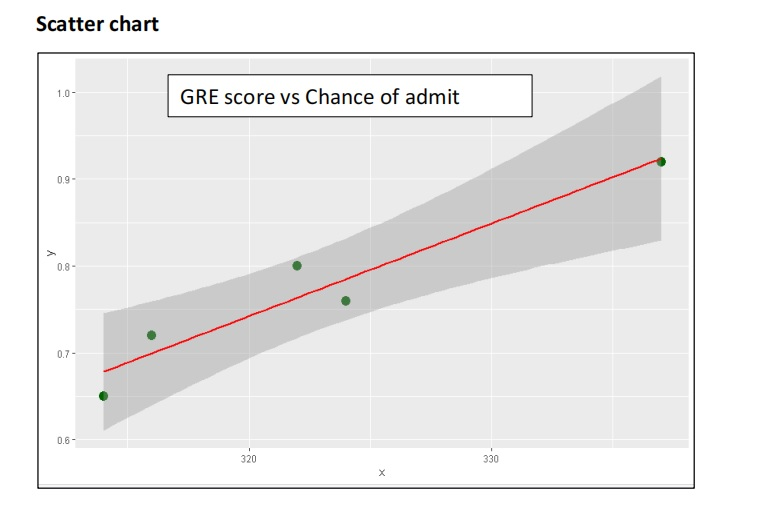Figure 6 : Scatterplot of top 5 Records in University Admission Dataset along with linear line

### Step 2: Calculating the Residuals

Now that we know there is a linear relationship between x and y, the system looks for a best fit regression line which minimise the residuals. Residuals are deviations of the actual data points from the regression line. The best fit line will have minimum residuals.

The regression line is sometimes called the "line of best fit" or the "best fit line". Since it "best fits" the data, the line passes through the mean of x and mean of y called the centroid.

GRE score ( X ) Chance of Admit ( Y )
Deviation GRE score
(Xi-mean(Xi))
Deviation Chance of Admit
(Yi-mean(Yi))
337
0.92
14.4
0.15
324
0.76
1.4
-0.01
316
0.72
-6.6
-0.05
322
0.8
-0.6
0.03
314
0.65
-8.6
-0.12
Mean (Xi)
Mean (Yi)

322.6
0.77

Figure 7 : Manual Computations for the Linear Regression LineFigure 8 : Graph showing Computation of residuals in Linear Regression

### Step 3: Calculating the Slope

Calculate the slope of the line using the following equation: -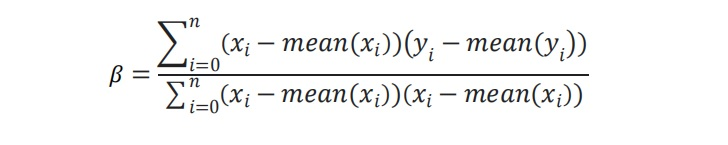Figure 9 : Table of University Admission Dataset beta compuation

ß = 3.49/327.2

= 0.010666259

As per the equation
y = ß*x +c
By substituting mean(x) and mean(y) in the equation, we get the value of the intercept (c)

c = 0.77 - 0.010666259 * 322.6

=  - 2.670935208

Final equation:
Y = 0.010666259x - 2.670935208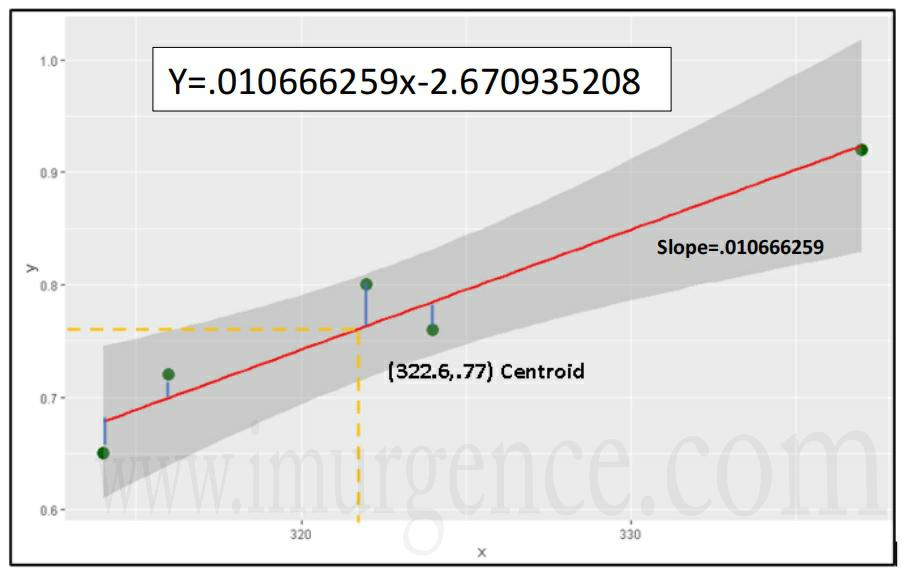Figure 10 : Regression line in University Admission Dataset along with the slope represenation

### Step 4 : Calculating the estimated value of y (chance of admit) using test dataset

GRE Score
Chance of
320
?
325
?

Y= 0.010666259 * 320 - 2.670935208

Y= 0.742267672

Y= 0.010666259 * 325 - 2.670935208

Y= 0.795598967

## What is R Square and how do you interpret R Squared in regression analysis?

R Square is also known as coefficient of determination and is a measure of the goodness of fit of the regression line to existing data points. The value of R Square ranges from 0 to 1.

If R Square is 1 then all the data points fall perfectly on the Regression line.

If R Square is 0, the regression line is horizontal. The predictor p does not account for any variation in the target variable y.

If R Square is between 0 and 1, the predictor p accounts for R2 *100 percent variation in the target variable y.

R square is represented by the following formula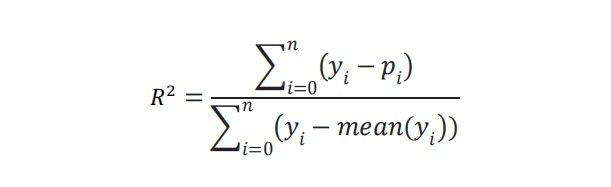Where,
yis the target or response variable in the test dataset and pi is the predicted response variable. R - square is calculated in the training dataset to understand the accuracy of the model.

## Implementing Linear Regression from scratch in Python

import pandas as pd
import the dataset
```df_admit=pd.read_csv("c:/csv/admission_predict.csv")
```
Extracting the first 5 records

df1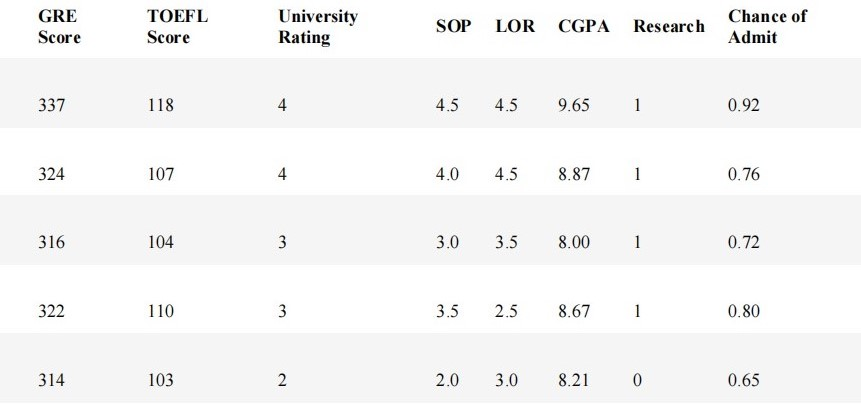Selecting the GRE Score and the Chance of admit column
df1=df1.iloc[:,[1,7]]
df1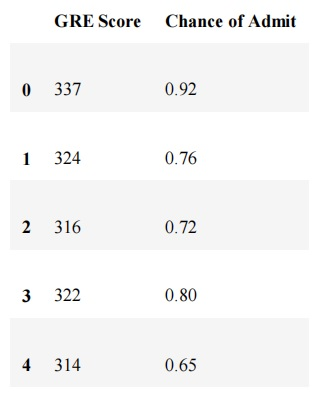setting GRE score as X vector
df1_x=df1.iloc[:,]
df1_x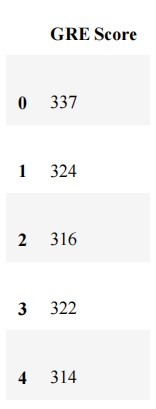converting object to numeric column
x=df1_x.iloc[:,0]
x=pd.to_numeric(x)

setting Chance of admit as Y vector
df1_y=df1.iloc[:,]
df1_y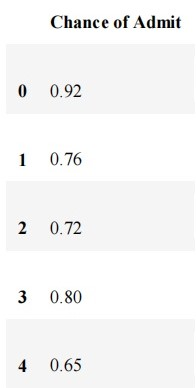converting object to numeric column
y=df1_y.iloc[:,0]
import numpy as np

calculating the mean of x and y vectors
df1_x_mean=np.mean(df1_x)
df1_x_mean

df1_y_mean=np.mean(df1_y)
df1_y_mean

calculating the deviation in x
deviation_x=[]
for i in x:
deviation_x.append(i-df1_x_mean)

deviation_x

calculating the deviation in y
deviation_y=[]
for i in y:
deviation_y.append(i-df1_y_mean)

deviation_y

calculating the product of deviations
```product_deviation=np.array(deviation_x)*np.array(deviation_y)

product_deviation

Sum_product_deviation=np.sum(product_deviation)
Sum_product_deviation

```
calculating the square of deviation of x and y
```Sq_x_deviation=np.array(deviation_x)**2

Sq_x_deviation

Sum_Sq_x_deviation=np.sum(Sq_x_deviation)
Sum_Sq_x_deviation

```
calculating the regression coefficient, the slope of regression line
```Regression_coefficient=Sum_product_deviation/Sum_Sq_x_deviation

Regression_coefficient

```
calculating the intercept
```intercept=np.float(df1_y_mean)-(Regression_coefficient*np.float(df1_x_mean))

intercept

```
calculating the predicted value of y from test data
```predicted_y1=Regression_coefficient*test_data+intercept

predicted_y1

predicted_y2=Regression_coefficient*test_data+intercept
predicted_y2

```
Implementing linear regression with SKLearn library

from sklearn.linear_model import LinearRegression
model1=LinearRegression()

#training the model with X as feature and Y as target

model1=model1.fit(df1_x,df1_y)

printing the regression coefficient or the slope of the regression line

print(model1.coef_)

printing the intercept

print(model1.intercept_)

creating the test dataset

x_test=pd.DataFrame({"GRE Score":[320,325]})

Predicting the value of Y (Chance of admit)

p=model1.predict(x_test)
p

Calculating R2_score

from sklearn.metrics import r2_score

The target variable of the test dataset is unknown so we need to use the training dataset

p_tr=model1.predict(df1_x)
r2_score(df1_y,p_tr)

We have done this implemenation on the top 5 records of the dataset. As a next step you should implement the same on the complete data set.